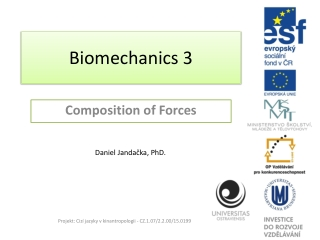DownloadDownload PresentationBiomechanics 3

# Biomechanics 3

Télécharger la présentation## Biomechanics 3

- - - - - - - - - - - - - - - - - - - - - - - - - - - E N D - - - - - - - - - - - - - - - - - - - - - - - - - - -
##### Presentation Transcript

1. Biomechanics3 CompositionofForces Daniel Jandačka, PhD. Projekt: Cizí jazyky v kinantropologii - CZ.1.07/2.2.00/15.0199

2. The net force is a vector produced when two or more forces act on a single object. It is calculated by vector addition of the force vectors acting on the object. Squat Jump of Shot Putterwith barbell Measured at Human Motion Diagnostic Center University of Ostrava Resultant (Net) Force = Blue Rreaction – Red Gravitational

3. Measured at Human Motion Diagnostic Center University of Ostrava 100 N 200 N 400 N Forces acting in one line can be added by algebraic sum. Example A coach is assisting his charge in bench press with a barbell of 100 kg. The coach is acting on the barbell with the force of 70 N and the athlete with the force of 920 N in upward direction. Will they manage to lift the barbell? What is the resultant force acting on the barbell? Solution Weight force of the barbell can be calculated as: FG = mg = 100 ⋅ 9,81 = 981 N. Let us assume that upward direction of the force is a positive direction, therefore: F = 70 N + 920 N + (−981 N) = 9 N. The resultant force F is 9 N and the athlete, with a little help from his coach, will manage to lift the barbell.

4. ConcurrentForces Resultant force vector is the diagonal of the rectangle created by both horizontal and vertical force and their parallel lines. Přesněji můžeme popsat působení síly úhlem mezi výslednou silou a vertikální nebo horizontální přímkou.

5. TrigonometricTechnique Example Vertical reaction force of the Earth (normal contact force), acting on a runner’s foot, has the magnitude of FRA = 2200 N; friction force is acting backward and its magnitude is FRB = 500 N. What is the direction and the magnitude of the resultant force FRC Figure Landing in running. The blue arrow represents the resultant reaction force FRC. Black arrows represent friction component of the reaction force FRB and vertical component of the reaction force FRA, respectively, both acting on the foot. Solution To calculate the resultant force, we will use the Pythagoras’ theorem: FRA2 + FRB2 = FRC2FRC2 = 22002 + 5002FRC = 2256 N. Andarctangent function (arctgFRC / FRB) to calculate the angle between the resultant and the horizontal force: The magnitude of the resultant force FRC is 2256 N and its angle with horizontal planeis α = 77,5°.

6. The magnitude of the resultant force from two perpendicular forces can be calculated with the use of the Pythagoras’ theorem, its direction can be calculated with the use of trigonometry.

7. ResolutionofForces Resolution of forces allows us to analyze causes of motion separately in vertical, mediolateral, and anteroposterior directions. Figure Resolution of reaction force, acting on human foot when walking, into three component forces. Solid line represents components of the reaction force acting on left foot; dashed line represents components of the reaction force acting on right foot. Vertical component forces are marked with blue, anteroposteriorcomponent forces are marked with black and mediolateral component forces are marked with grey. Resolution of Forces allows to better understand human neuromuscular functions in many motor tasks.

8. Equilibrium Statics is the branch of mechanics studying forces that act on bodies in static or dynamic equilibrium. Dynamicequilibrium is a state where bodies are moving at a constant velocity (rectilinear motion) Static equilibrium is a state where bodies are at rest In both cases the sum of the forces acting on them is zero.

9. Free body diagram pictures a body of interest (athlete) and external forces acting on it. FigureFree body diagram. FR is the sum of reaction forces acting on both legs and FG is gravitational force acting on athlete’s body. Note In reality reaction forces originate in the place of contact between the feet and the ground. However, for the sake of this free body diagram we can plot the resultant reaction force whose point of application is in so called „centre of pressure”, i.e. away from the place of contact between the feet and the ground.

10. Static Analysis If only two forces act on a body in the state of either static or dynamic equilibrium, they have equal magnitude but opposite direction. Example Weightlifter with the weight of 70 kg has lifted a barbell with the weight of 90 kg and is holding it above his head. As long as he is holding the barbell, both bodies (weightlifter and barbell) are in static equilibrium. What is the force that must act on the weightlifter’s feet to keep him in static equilibrium?

11. Thank you for your attention Projekt: Cizí jazyky v kinantropologii - CZ.1.07/2.2.00/15.0199